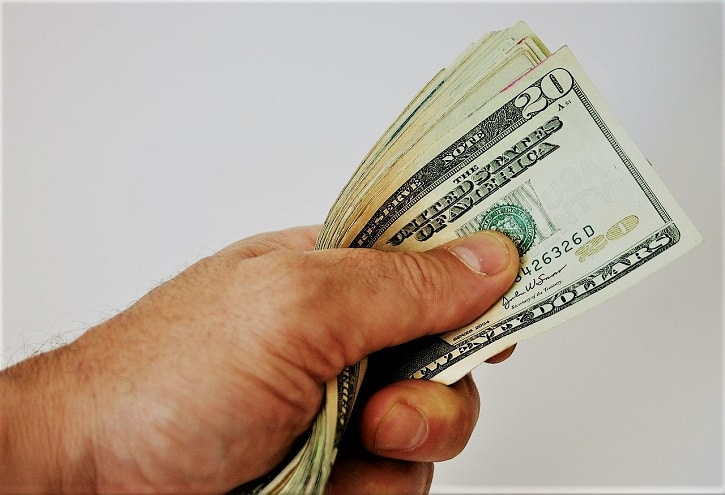# How do Payday Loans Work OnlineSharing is caring!

# How do Payday Loans Work Online## What are Payday Loans?

Payday loans are small loans up to \$1500, that are intended to be repaid in sixty two days or less. These loans are given by licensed lenders. To qualify for a payday loan, is much simpler than a loan you would normally get through a bank.

To qualify for a payday loan you need to:

• Be 18 years old
• Reside legally in the United States
• You must be employed for at leas 90 days in a row
• A bank account, not overdrawn
• An email and a telephone number
• and meeting the minimum income requirements, based on the amount of money you will borrow.

Now, you might be wondering how do payday loans work online. There are websites who can match you with possible lenders. These websites have huge databases of possible licensed lenders and they can much your inquiry with the best possible match.

Usually, you can complete an online form – a process that takes only a couple of minutes. The process is absolutely safe and completely free. All these websites ask is for the required info in order to match you with a possible lender.

Once you found the lender who is willing to borrow you money, you are going to examine the loan agreement details. Then you have to provide an electronic signature to close the contract. After this step is done, the lender deposits the money you requested to your bank account. Normally, you’ll get your money in by the end of the next day.

## How Do You Calculate The Interest Rate of a Payday Loan

A payday loan generally refers to a short-term loan of \$1,500 or less with a term of 62 days or less.

Normally when you borrow money, safe for using a credit card or for your mortgage, the interest rate you are being charged is known.

For example, if you were borrowing money to buy your first house at the beginning of 2015 you probably know you could get an interest rate of less than three percent. You might clearly know that your credit card provider charges a fixed annual interest rate of say nineteen percent, but payday loans are advertised a little differently. Instead of telling you the rate first, they tell you the dollar amount of borrowing.

For example, it’s common to see ads that will say borrow three hundred dollars for two weeks for \$69. What you might not realize is that a cost of borrowing \$300 for two weeks for \$69 is the equivalent of a 599.64 percent interest rate.

Here’s how to figure that rate out:

Take the cost of borrowing which is \$69, and divide that by the amount borrowed which is \$300.

This gives you the interest rate for the period of the loan.

This simple interest rate is twenty-three percent.

Now you have to convert that into a simple annual rate.

Payday loans are not allowed to be compounded continuously. To do that, we have to figure out how many of these two week period fit into a year.

Your first instinct is to think «Well, there are 52 weeks in a year, so that’s 26 two-week periods. Multiply 23% – for two week period – by 26 to get five hundred ninety percent. But that’s not actually the exact formula used.

Non leap years have 365 days, but note that 52 weeks x 7 days per week is 364. So, the exact formula requires using a

more precise number. To figure out how many two-week period there are in a year.

We divide 365 days by 14 days to get 26.0714.

We then multiply this by the interest rate for two weeks of 23% to get a simple annual percentage rate of 599.64 percent.

Let’s see another example:

Let’s say you were borrowing \$200 with a cost of borrowing of forty-four dollars and you’re only borrowing for 10 days.

The rate for the period is forty-four dollars divided by two hundred dollars or 22-percent.

The period is 10 days.

To figure out the simple annual percentage rate, we need to multiply 22 percent per ten days by the number of ten day periods in a year, which is given by 365 days divided by ten days or 36.5.

Now, we simply take twenty two percent and multiply by 36.5. This gives us a simple annual percentage rate of 803 percent. So borrowing two hundred dollars for forty four dollars over ten days has an annual percentage rate of 803 percent.

And now you know how to calculate the annual interest rate equivalent on a short term payday loan before you take a payday loan. Click here to get a us credit loan from a trusted source. Make sure you do your calculations first, if you need to understand the amount of interest you’ll be charged per year.

Of course, payday loans are not meant to last for a year. You are advised to repay your payday loans the soonest possible and take these kind of loans whenever you are in a real need.

If you liked this article, please share it with your friends. We are sure they would love to learn how do payday loans work online. On the other hand, by sharing this article, is like saying thank you to the author.

Image info: By Gregory F. Maxwell <gmaxwell@gmail.com> PGP:0xB0413BFAOwn work (Original caption: “By Uploader”), GFDL 1.2, Link

Sharing is caring!